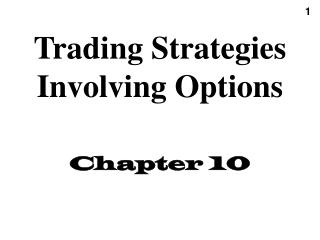DownloadDownload PresentationTrading Strategies Involving Options Chapter 10

# Trading Strategies Involving Options Chapter 10

Télécharger la présentation## Trading Strategies Involving Options Chapter 10

- - - - - - - - - - - - - - - - - - - - - - - - - - - E N D - - - - - - - - - - - - - - - - - - - - - - - - - - -
##### Presentation Transcript

2. Positions in an Option & the Underlying • Buy or sell call • Buy or sell put • buy or short sell stock • buy (lend at) or sell (borrow at) risk-free bond (rate)

3. Trading Strategies Involving Options • Take a position in the option & the underlying • Spread: Position in 2 or more options of the same type • Combination: Position in a mixture of calls & puts

4. Payoff for Put Option x Cost = p buy ST X sell -X Cost = -p

5. Payoff for Call Option x Cost = c buy ST X sell Cost = -c

6. Payoff for Stock buy Cost = S ST sell Cost = -S

7. Payoff for Investing or Borrowing PV(X) in Risk-Free Bond Cost = Xe-rT X buy ST Cost = -Xe-rT sell -X

8. Method • Draw payoff pattern for each position • Add patterns together to obtain payout pattern • Determine cost of portfolio • Subtract cost of portfolio to obtain profit pattern

9. Write a Covered Call buy stock X Cost = S - c ST X sell call

10. Profit Pattern for Covered Call Cost = S - c X X-S+c ST X -S+c X-S+c

11. Payoff for Protective Put Cost = S + p x ST X

12. Profit Pattern Protective Put Cost = S + p x X ST X-S-p S+p

13. Bull Spread • Buy 1 call and sell 1 call at higher strike X2-X1 buy Cost = c1 - c2 > 0 ST X1 X2 sell

14. Profit Pattern for Bull Spread X2-X1 -c1+c2 ST -c1+c2

15. Bear Spread • Sell 1 call and buy 1 call at higher strike buy Cost = c1 - c2 < 0 ST X2 X1 sell X2-X1

16. Profit Pattern for Bear Spread Cost = c1 - c2 < 0 ST X2 X1

17. Buy a Straddle • Buy 1 call and 1 put at same strike X Cost = c + p payout profit X -p-c X-p-c X+p+c

18. Butterfly Spread • Buy 1 call at X1, sell 2 calls at X2, buy 1 call at X3 m = +1 m = -1 m = 0 X2 X1 X3 m = - 2 Cost = c1 - 2c2 + c3

19. Butterfly Spread • Buy 1 call, sell 2 calls, buy 1 call X2-X1 payoff cost profit Cost = c1 - 2c2 + c3

20. Bull Spread Using Calls • Figure 10.2 Profit X1 X2 ST

21. Figure 10.3 Profit X1 X2 ST Bull Spread Using Puts

22. Figure 10.4 Profit X1 X2 ST Bear Spread Using Calls

23. Figure 10.5 Profit X1 X2 ST Bear Spread Using Puts

24. Figure 10.6 Profit X1 X2 X3 ST Butterfly Spread Using Calls

25. Figure 10.7 Profit X1 X2 X3 ST Butterfly Spread Using Puts

26. Figure 10.10 Profit X ST A Straddle Combination

27. Strip & Strap • Figure 10.11 Profit Profit X ST X ST Strip Strap

28. Figure 10.12 Profit X1 X2 ST A Strangle Combination